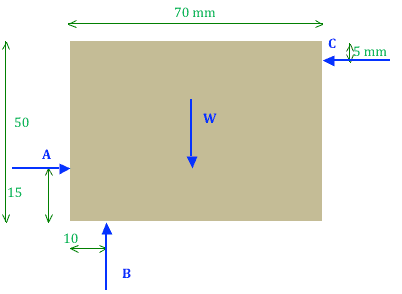# Moment Equilibrium in terms of box weight

• Blobikins

## Homework Statement

The board shown below is being held in equilibrium in the vertical plane by three smooth rods at A, B, and C
Determine the magnitudes of forces at A, B, and C in terms of the weight of the body, W.

## Homework Equations

Moment = f*r

Here's a diagram## The Attempt at a Solution

I'm assuming the point of rotation is in the middle, at 'W'

So, B will be 25mm away, pushing clockwise
A, 10 mm away pushing clockwise
C 20 mm away pushing CCW.

I don't exactly get what they mean by 'In terms of the weight of the body 'W'" , because W is outside of the input section, and it only accepts numbers into the blank. Thank you.

•